Published: 26 June 2023

# Influence of the fractional-order strain on an infinite material with a spherical cavity under Green-Naghdi hyperbolic two-temperature thermoelasticity theory

Hamdy M. Youssef1
Abdulrahman A. Alghamdi2
1Mechanical Engineering Department, College of Engineering and Islamic Architecture, Umm Al-Qura University, Makkah, 21955, Saudi Arabia
2Mathematics Department, College of Applied Sciences, Umm Al-Qura University, Makkah, 21955, Saudi Arabia
Corresponding Author:
Hamdy M. Youssef
Editor's Pick
Views 61

#### Abstract

In this work, a novel mathematical model of thermoelastic, homogenous, isotropic, and infinite medium with a spherical cavity has been constructed. Under the hyperbolic two-temperature Green-Naghdi theory of thermoelasticity type-I and type-III with fractional-order strain, the governing equations have been established. The bounding surface of the cavity has been thermally loaded by a ramp-type heat and is connected to a rigid foundation which prevents volumetric strain. Different values of the fractional-order and two-temperature parameters have shown numerical results for the dynamical and conductive temperature increment, strain, displacement, and average of principal stresses, which are graphically applicable to all the functions studied. The fractional-order parameter has significant effects on stress and strain distributions, while it has a limited effect on the dynamical and conductive temperatures increment. The hyperbolic two-temperature parameter has significant effects on all studied functions based on Green-Naghdi models of type-1 and type-II. Moreover, the ramp-time heat parameter has a significant impact on all the studied functions under all the studied models of thermoelasticity.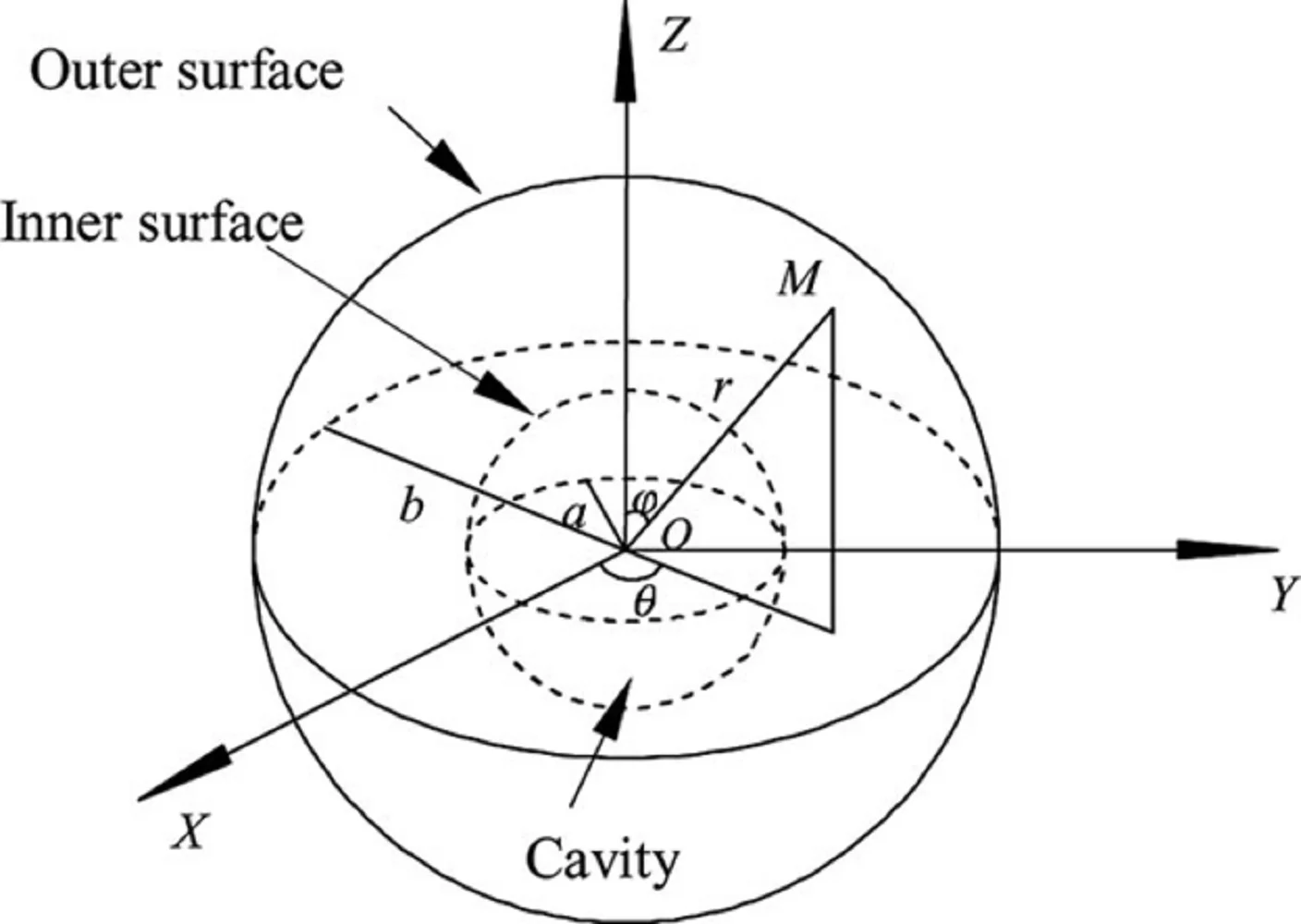#### Highlights

• This research examined how skin tissue responds to a steady heat flux caused by a thermo-electrical shock on the bounding plane in terms of temperature reaction and response.
• The fractional-order strain parameter significantly affects strain and stress.
• The voltage, resistance, and dual-lag time values significantly affect the distribution of temperature increments and the propagation of thermal waves through skin tissue.
• The Tzou model is the most effective model that accurately predicts the physical behaviour of skin tissue.
• Green-Naghdi type-I thermal wave propagation profiles are smaller than type-III.
• Strain reduces mechanical wave propagation profile with a fractional-order and hyperbolic two-temperature heat conduction model.

## 1. Introduction

How to find a precise model that accurately represents the behaviour of thermoelastic materials is the main issue of the topic. Researchers and writers have created several models that depict how waves are transferred through solids and thermoelastic materials. However, not all of these models are successful because one of the criteria for a good model is to reproduce experimental findings with waves of mechanical and thermal propagation moving at a finite speed. No one field of study can be used to discuss thermomechanical transition models that apply to elastic materials .

Based on the idea of fractional calculus, some fresh thermoelastic models were presented. The first model describing the behaviour of the material was created by Magin and Royston using the fractional deformation derivative . The derivative's zero order is a Hookean solid, while it's one order is a Newtonian fluid. The split order of thermoviscoelastic materials and the intermediate heat exchange spectrum .

Youssef introduced a different new theory of global thermoelasticity based on the fractional-order strain. It is believed that the connection between stress and strain is a novel and distinctive contribution to Duhamel-Neumann's theory . Youssef has solved the type-II problems of Biot, Lord-Shulman, Green-Lindsay, and thermoelasticity in one dimension with fractional sequence strain .

Based on two distinct conductive and dynamic temperatures, Chen and Gurtin created the thermoelasticity model. The relationship between the temperature difference and the heat source . Warren and Chen looked at the propagation of waves in the two-temperature thermoelastic theory . However, there won't be any research on that theory before Youssef updates it and develops a two-temperature generalized thermostat model . Youssef and many other authors applied this concept in several applications and enquiries [7-13]. Youssef and El-Bary validated the two-temperature generalized thermoelasticity model, although it does not give a fixed speed for thermal wave propagation . To replace this model, Youssef and El-Bary created the hyperbolic two-temperature generalized thermoelasticity model, which is based on new thermal conductivity principles . Youssef proposed that the difference between conductive temperature and dynamic temperature acceleration in that model be proportional to the heat supply. In this model, the rate of thermal wave propagation is constrained. Youssef found solutions to several uses of the infinite thermoelastic spherical media [15-18]. In the limitless media, Mukhopadhyay and Kumar investigated the universal thermoelastic interactions with the cavity . Many authors used spherical cavities to solve problems involving thermoelastic mediums [20-29].

In the current work, with a spherical cavity, a novel mathematical model of a thermoelastic, homogenous, isotropic, and infinite medium will be developed. The governing equations will be constructed for the hyperbolic two-temperature Green-Naghdi theory of thermoelasticity type-1 and type-III based on fractional-order strain consideration. A ramp-type heat will thermally load the cavity's boundary, and it is attached to a rigid foundation to avoid volumetric strain. Different fractional-order and two-temperature parameter values will be produced numerical findings for the strain, displacement, average principle stresses, and dynamical and conductive temperature increment, which are visually relevant to all the functions under study.

## 2. The problem formulation

Consider a perfect, thermoelastic, conducting, and isotropic body with a spherical cavity that occupies the region $\xi =\left\{\left(r,\psi ,\varphi \right):a\le r<\infty ,0\le \psi \le 2\pi ,0\le \varphi <2\pi \right\}$. We use a spherical coordinative system $\left(r,\psi ,\varphi \right)$ that displays the radial coordinate, co-latitude, and longitude of a spherical system, without any forces on the body and initially calming where r is the sphere radius, as in Fig. 1. When there are no latitude and longitudinal variance is the symmetry condition fulfilled. Both state functions depend on the distance and time of the radius.

Fig. 1The isotropic homogeneous thermoelastic solid sphere with a spherical cavity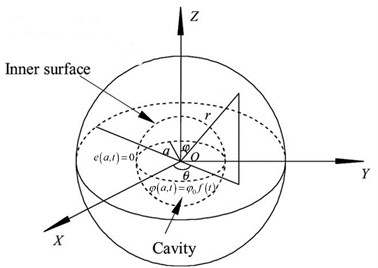We note that due to spherical symmetry, the displacement components have the form:

1
$\left({u}_{r},{u}_{\psi },{u}_{\varphi }\right)=\left(u\left(r,t\right),0,0\right).$

The equations of motion [3, 13]:

2
$\rho \stackrel{¨}{u}=\left(\lambda +2\mu \right)\left(1+{\tau }^{\alpha }{D}_{t}^{\alpha }\right)\frac{\partial e}{\partial r}-\gamma \frac{\partial {T}_{D}}{\partial r}.$

The constitutive equations with damage mechanics variable [3, 13]:

3
${\sigma }_{rr}=\left(1+{\tau }^{\alpha }{D}_{t}^{\alpha }\right)\left(2\mu {e}_{rr}+\lambda e\right)-\gamma \left({T}_{D}-{T}_{0}\right),$
4
${\sigma }_{\psi \psi }=\left(1+{\tau }^{\alpha }{D}_{t}^{\alpha }\right)\left(2\mu {e}_{\psi \psi }+\lambda e\right)-\gamma \left({T}_{D}-{T}_{0}\right),$
5
${\sigma }_{\varphi \varphi }=\left(1+{\tau }^{\alpha }{D}_{t}^{\alpha }\right)\left(2\mu {e}_{\varphi \varphi }+\lambda e\right)-\gamma \left({T}_{D}-{T}_{0}\right),$
6
${\sigma }_{r\varphi }={\sigma }_{\varphi \psi }={\sigma }_{r\psi }=0.$

The strain components are:

7
${e}_{rr}=\frac{\partial u}{\partial r},{e}_{\psi \psi }={e}_{\varphi \varphi }=\frac{u}{r},$

and

8
${e}_{r\varphi }={e}_{\varphi \psi }={e}_{r\psi }=0,$

where $e$ is the cubical dilatation and is given by:

9
$e={e}_{rr}+2{e}_{\varphi \varphi }=\frac{\partial u}{\partial r}+\frac{2u}{r}=\frac{1}{{r}^{2}}\frac{\partial \left({r}^{2}u\right)}{\partial r}.$

The hyperbolic two-temperature heat conduction equations take the forms [3, 13, 14].

The heat conduction equations which have been proposed by Green-Naghdi take the following form :

10
$\left(\frac{\partial }{\partial t}+\stackrel{~}{K}\right){\nabla }^{2}{T}_{C}=\frac{{\partial }^{2}}{\partial {t}^{2}}\left(\frac{\rho {C}_{v}}{K}{T}_{D}+\frac{\gamma {T}_{0}}{K}\left(1+{\tau }^{\alpha }{D}_{t}^{\alpha }\right)e\right).$

The unified Eq. (6) could be used for the two types of Green-Naghdi theories as follows:

The setting $\stackrel{~}{K}=0$ represents the Green-Naghdi type-I model.

The setting $\stackrel{~}{K}={K}^{\mathrm{*}}/K$ represents the Green-Naghdi type-III model, where ${K}^{\mathrm{*}}=\left(\lambda +2\mu \right){C}_{v}/4$ is the characteristic of Green-Naghdi theory, $K$ is the usual thermal conductivity, and the unit of the quantity $\left(\stackrel{~}{K}\right)$ is ${\mathrm{s}}^{-1}$ and:

11
${\stackrel{¨}{T}}_{C}-{\stackrel{¨}{T}}_{D}={c}^{2}{\nabla }^{2}{T}_{C},$

where $c$ (m/s) is the hyperbolic two-temperature parameter , and ${\nabla }^{2}=\frac{1}{{r}^{2}}\frac{\partial }{\partial r}\left({r}^{2}\frac{\partial }{\partial r}\right)$.

The Riemann – Liouville fractional integral ${I}^{\alpha }f\left(t\right)$ description is used in the above equations written in a convolution-type form [3, 30]:

12
${I}^{\alpha }f\left(t\right)=\frac{1}{\mathrm{\Gamma }\left(\alpha \right)}{\int }_{0}^{t}{\left(t-\upsilon \right)}^{\alpha -1}f\left(\upsilon \right)d\upsilon ,t>0,\mathrm{}\mathrm{}\mathrm{}\alpha >0,$

that provides Caputo with the form of fractional derivatives:

13
${D}_{t}^{\alpha }f\left(t\right)={I}^{-\alpha }f\left(t\right)=\left\{\begin{array}{ll}\frac{1}{\mathrm{\Gamma }\left(1-\alpha \right)}{\int }_{0}^{t}\frac{f\left(\xi \right)}{{\left(t-\xi \right)}^{\alpha }}d\xi ,& 0<\alpha <1,\\ f\left(t\right),& \alpha =0,\\ {f}^{\text{'}\left(t\right)},& \alpha =1.\end{array}\right\$

We consider that $\phi =\left({T}_{C}-{T}_{0}\right)$ and $\theta =\left({T}_{D}-{T}_{0}\right)$are the conductive and dynamical temperature increments, respectively. Then the Eqs. (2)-(5), (10) and (11) take the forms:

14
$\rho \stackrel{¨}{u}=\left(\lambda +2\mu \right)\left(1+{\tau }^{\alpha }{D}_{t}^{\alpha }\right)\frac{\partial e}{\partial r}-\gamma \frac{\partial \theta }{\partial r},$
15
$\left(\frac{\partial }{\partial t}+\stackrel{~}{K}\right){\nabla }^{2}\phi =\frac{{\partial }^{2}}{\partial {t}^{2}}\left(\frac{\rho {C}_{v}}{K}\theta +\frac{\gamma {T}_{0}}{K}\left(1+{\tau }^{\alpha }{D}_{t}^{\alpha }\right)e\right),$
16
$\stackrel{¨}{\phi }-\stackrel{¨}{\theta }={c}^{2}{\nabla }^{2}\phi ,$
17
${\sigma }_{rr}=\left(1+{\tau }^{\alpha }{D}_{t}^{\alpha }\right)\left(2\mu {e}_{rr}+\lambda e\right)-\gamma \theta ,$
18
${\sigma }_{\psi \psi }=\left(1+{\tau }^{\alpha }{D}_{t}^{\alpha }\right)\left(2\mu {e}_{\psi \psi }+\lambda e\right)-\gamma \theta ,$
19
${\sigma }_{\varphi \varphi }=\left(1+{\tau }^{\alpha }{D}_{t}^{\alpha }\right)\left(2\mu {e}_{\varphi \varphi }+\lambda e\right)-\gamma \theta ,$

The Eq. (14) can be re-written to be in the form:

20
$\frac{\rho }{\left(\lambda +2\mu \right)}\stackrel{¨}{e}=\left(1+{\tau }^{\alpha }{D}_{t}^{\alpha }\right){\nabla }^{2}e-\frac{\gamma }{\left(\lambda +2\mu \right)}{\nabla }^{2}\theta .$

The following non-dimensional variables are used for convenience [8, 12, 13]:

21
$\left\{r\text{'},u\text{'},a\text{'}\right\}={c}_{o}\eta \left\{r,u,a\right\},\left\{t\text{'},\tau \text{'}\right\}={c}_{o}^{2}\eta \left\{t,\tau \right\},\stackrel{~}{K\text{'}}=\frac{\stackrel{~}{K}}{{c}_{o}^{2}\eta },$
$\left\{\theta \text{'},\phi \text{'}\right\}=\frac{1}{{T}_{0}}\left\{\theta ,\phi \right\},{\sigma }^{\text{'}}=\frac{\sigma }{\lambda +2\mu }.$

Then, we obtain:

22
$\stackrel{¨}{e}=\left(1+{\tau }^{\alpha }{D}_{t}^{\alpha }\right){\nabla }^{2}e-\epsilon {\nabla }^{2}\theta ,$
23
$\left(\frac{\partial }{\partial t}+\stackrel{~}{K}\right){\nabla }^{2}\phi =\frac{{\partial }^{2}}{\partial {t}^{2}}\left(\theta +{\epsilon }_{1}\left(1+{\tau }^{\alpha }{D}_{t}^{\alpha }\right)e\right),$
24
$\stackrel{¨}{\theta }=\stackrel{¨}{\phi }-{\stackrel{~}{c}}^{2}{\nabla }^{2}\phi ,$
25
${\sigma }_{rr}=\left(1+{\tau }^{\alpha }{D}_{t}^{\alpha }\right)\left({\beta }^{2}{e}_{rr}+\left(1-{\beta }^{2}\right)e\right)-\epsilon \theta ,$
26
${\sigma }_{\psi \psi }=\left(1+{\tau }^{\alpha }{D}_{t}^{\alpha }\right)\left({\beta }^{2}{e}_{\psi \psi }+\left(1-{\beta }^{2}\right)e\right)-\epsilon \theta ,$
27
${\sigma }_{\varphi \varphi }=\left(1+{\tau }^{\alpha }{D}_{t}^{\alpha }\right)\left({\beta }^{2}{e}_{\varphi \varphi }+\left(1-{\beta }^{2}\right)e\right)-\epsilon \theta .$

The prims have been deleted for simplicity.

## 3. Problem formulation in the Laplace transform domain

The Laplace transform will be applied which is defined as follows:

28
$\stackrel{-}{h}\left(r;s\right)={\int }_{0}^{\infty }{e}^{-st}\stackrel{-}{h}\left(r;t\right)dt,$

where the inversion of the Laplace transform may be calculated numerically by the following iteration:

29
$h\left(r;t\right)={L}^{-1}\left[\stackrel{-}{h}\left(r;s\right)\right]\approx \frac{{e}^{\kappa t}}{2t}\stackrel{-}{h}\left(r;\kappa \right)+\frac{{e}^{\kappa t}}{t}\mathrm{R}\mathrm{e}{\sum }_{n=1}^{N}{\left(-1\right)}^{n}\stackrel{-}{h}\left(r;\frac{\kappa t+in\pi }{t}\right),$

where $Re$ denotes the real part, while $i$ defines the unit imaginary number. Numerous numerical tests have been conducted to determine if the value of $\kappa$ may meet the relation $\kappa t\approx 4.7\mathrm{}$[31, 32].

The Laplace transform of the fractional derivative is defined as :

30
$L\left\{{D}_{t}^{\alpha }f\left(t\right)\right\}={s}^{\alpha }\stackrel{-}{f}\left(s\right)-{D}_{t}^{\alpha }{I}^{1-\alpha }f\left({0}^{+}\right),0<\alpha <1.$

We assume the following initial conditions:

31
${D}_{t}^{\alpha }{I}^{1-\alpha }\left\{e\left(r,{0}^{+}\right),\phi \left(r,{0}^{+}\right),\theta \left(r,{0}^{+}\right)\right\}=0,0<\alpha <1$
32
${{e\left(r,t\right)|}_{t=0}=\theta {\left(r,t\right)}_{t=0}=\phi {\left(r,t\right)}_{t=0}=\frac{\partial e\left(r,t\right)}{\partial t}|}_{t=0}={\frac{\partial \theta \left(r,t\right)}{\partial t}|}_{t=0}={\frac{\partial \phi \left(r,t\right)}{\partial t}|}_{t=0}=0.$

Then, we obtain:

33
${s}^{2}\stackrel{-}{e}=\left(1+{\tau }^{\alpha }{s}^{\alpha }\right){\nabla }^{2}\stackrel{-}{e}-\epsilon {\nabla }^{2}\stackrel{-}{\theta },$
34
$\left(s+\stackrel{~}{K}\right){\nabla }^{2}\stackrel{-}{\phi }={s}^{2}\stackrel{-}{\theta }+{\epsilon }_{1}{s}^{2}\left(1+{\tau }^{\alpha }{s}^{\alpha }\right)\stackrel{-}{e},$
35
${s}^{2}\stackrel{-}{\theta }={s}^{2}\stackrel{-}{\phi }-{\stackrel{~}{c}}^{2}{\nabla }^{2}\stackrel{-}{\phi },$
36
${\stackrel{-}{\sigma }}_{rr}=\left(1+{\tau }^{\alpha }{s}^{\alpha }\right)\left({\beta }^{2}{\stackrel{-}{e}}_{rr}+\left(1-{\beta }^{2}\right)\stackrel{-}{e}\right)-\epsilon \stackrel{-}{\theta },$
37
${\stackrel{-}{\sigma }}_{\psi \psi }=\left(1+{\tau }^{\alpha }{s}^{\alpha }\right)\left({\beta }^{2}{\stackrel{-}{e}}_{\psi \psi }+\left(1-{\beta }^{2}\right)\stackrel{-}{e}\right)-\epsilon \stackrel{-}{\theta },$
38
${\stackrel{-}{\sigma }}_{\varphi \varphi }=\left(1+{\tau }^{\alpha }{s}^{\alpha }\right)\left({\beta }^{2}{\stackrel{-}{e}}_{\varphi \varphi }+\left(1-{\beta }^{2}\right)\stackrel{-}{e}\right)-\epsilon \stackrel{-}{\theta }.$

Eliminating $\stackrel{-}{\theta }$ from the Eqs. (33)-(35), we obtain:

39
${\nabla }^{2}\stackrel{-}{e}-{\alpha }_{1}\stackrel{-}{e}={\alpha }_{2}{\nabla }^{2}\stackrel{-}{\phi }-{\alpha }_{3}{\nabla }^{4}\stackrel{-}{\phi },$
40
${\nabla }^{2}\stackrel{-}{\phi }={\alpha }_{4}\stackrel{-}{\phi }+{\alpha }_{5}\stackrel{-}{e},$

where:

${\alpha }_{1}=\frac{{s}^{2}}{\left(1+{\tau }^{\alpha }{s}^{\alpha }\right)},{\alpha }_{2}=\frac{\epsilon }{\left(1+{\tau }^{\alpha }{s}^{\alpha }\right)},{\alpha }_{3}=\frac{{\stackrel{~}{c}}^{2}\epsilon }{{s}^{2}\left(1+{\tau }^{\alpha }{s}^{\alpha }\right)},$
${\alpha }_{4}=\frac{{s}^{2}}{\left(s+\stackrel{~}{K}+{\stackrel{~}{c}}^{2}\right)},{\alpha }_{5}=\frac{{\epsilon }_{1}{s}^{2}\left(1+{\tau }^{\alpha }{s}^{\alpha }\right)}{\left(s+\stackrel{~}{K}+{\stackrel{~}{c}}^{2}\right)}.$

Eliminating $\stackrel{-}{e}$ from the Eqs. (38) and (39), we get:

41
$\left[{\nabla }^{4}-L{\nabla }^{2}+M\right]\stackrel{-}{\phi }=\left({\nabla }^{2}-{k}_{1}^{2}\right)\left({\nabla }^{2}-{k}_{2}^{2}\right)\stackrel{-}{\phi }=0.$

Eliminating $\stackrel{-}{\phi }$ from the Eqs. (39) and (40), we obtain:

42
$\left[{\nabla }^{4}-L{\nabla }^{2}+M\right]\stackrel{-}{e}=\left({\nabla }^{2}-{k}_{1}^{2}\right)\left({\nabla }^{2}-{k}_{2}^{2}\right)\stackrel{-}{e}=0,$

where $L=\frac{\left({\alpha }_{1}+{\alpha }_{4}+{\alpha }_{2}{\alpha }_{5}\right)}{\left(1+{\alpha }_{3}{\alpha }_{5}\right)}$, $M=\frac{{\alpha }_{1}{\alpha }_{4}}{\left(1+{\alpha }_{3}{\alpha }_{5}\right)}$, and $±{k}_{1},±{k}_{2}$ are the roots of the following characteristic equation:

43
${k}^{4}-L{k}^{2}+M=0.$

The general solutions of the Eqs. (41) and (42) must be bounded at infinity, thus, they take the following forms:

44
$\stackrel{-}{\phi }\left(r,s\right)={A}_{1}\frac{{e}^{-{k}_{1}r}}{r}+{A}_{2}\frac{{e}^{-{k}_{2}r}}{r},a\le r<\infty ,$
45
$\stackrel{-}{e}\left(r,s\right)={B}_{1}\frac{{e}^{-{k}_{1}r}}{r}+{B}_{2}\frac{{e}^{-{k}_{2}r}}{r},a\le r<\infty .$

From the Eq. (39), we obtain:

46
$\left({k}_{i}^{2}-{\alpha }_{4}\right){A}_{i}={\alpha }_{5}{B}_{i},i=1,2.$

Thus, we have:

47
$\stackrel{-}{e}\left(r,s\right)=\left({k}_{1}^{2}-{\alpha }_{4}\right){A}_{1}\frac{{e}^{-{k}_{1}r}}{{\alpha }_{5}r}+\left({k}_{2}^{2}-{\alpha }_{4}\right){A}_{2}\frac{{e}^{-{k}_{2}r}}{{\alpha }_{5}r},a\le r<\infty .$

To get the parameters ${A}_{1}$ and ${A}_{2}$, we must apply the boundary conditions, so we will consider that the surface of the cavity is subjected to a thermal loading with a function of time only as follows:

48
$\phi \left(a,t\right)={\phi }_{0}f\left(t\right).$

Moreover, we will consider that the surface of the cavity is connected to a rigid foundation which can stop any cubical deformation, i.e., we have:

49
$e\left(a,t\right)=0.$

After applying Laplace transform, we obtain:

50
$\stackrel{-}{\phi }\left(a,s\right)={\phi }_{0}\stackrel{-}{f}\left(s\right),$
51
$\stackrel{-}{e}\left(a,s\right)=0.$

By using the conditions Eqs. (50) and (51) into the Eqs. (44) and (47), we obtain the following system of linear equations:

52
${A}_{1}{e}^{-{k}_{1}a}+{A}_{2}{e}^{-{k}_{2}a}=a{\phi }_{0}\stackrel{-}{f}\left(s\right),$
53
$\left({k}_{1}^{2}-{\alpha }_{4}\right){A}_{1}{e}^{-{k}_{1}a}+\left({k}_{2}^{2}-{\alpha }_{4}\right){A}_{1}{e}^{-{k}_{2}a}=0.$

By solving the above system, we get:

54
${A}_{1}=\frac{\left({k}_{2}^{2}-{\alpha }_{4}\right)a{\phi }_{0}\stackrel{-}{f}\left(s\right)}{{k}_{2}^{2}-{k}_{1}^{2}}{e}^{{k}_{1}a},{A}_{2}=-\frac{\left({k}_{1}^{2}-{\alpha }_{4}\right)a{\phi }_{0}\stackrel{-}{f}\left(s\right)}{{k}_{2}^{2}-{k}_{1}^{2}}{e}^{{k}_{2}a}.$

Hence, we have the solutions in the Laplace transform domain as follows:

55
$\stackrel{-}{\phi }\left(r,s\right)=\frac{a{\phi }_{0}\stackrel{-}{f}\left(s\right)}{{k}_{2}^{2}-{k}_{1}^{2}}\left[\left({k}_{2}^{2}-{\alpha }_{4}\right)\frac{{e}^{-{k}_{1}\left(r-a\right)}}{r}-\left({k}_{1}^{2}-{\alpha }_{4}\right)\frac{{e}^{-{k}_{2}\left(r-a\right)}}{r}\right],a\le r<\infty ,$
56
$\stackrel{-}{e}\left(r,s\right)=\frac{\left({k}_{1}^{2}-{\alpha }_{4}\right)\left({k}_{2}^{2}-{\alpha }_{4}\right)a{\phi }_{0}\stackrel{-}{f}\left(s\right)}{{\alpha }_{5}\left({k}_{2}^{2}-{k}_{1}^{2}\right)}\left(\frac{{e}^{-{k}_{1}\left(r-a\right)}}{r}-\frac{{e}^{-{k}_{2}\left(r-a\right)}}{r}\right),a\le r<\infty .$

Now, we must determine the thermal loading function $f\left(t\right)$, so we will consider that the thermal loading function is a ramp-type heating which takes the form [7, 33, 34]:

57
$f\left(t\right)=\left\{\begin{array}{ll}\frac{t}{{t}_{0}},& 0

where ${t}_{0}$ is called the ramp-time heat parameter.

After applying the Laplace transform to the above equation, we obtain:

58
$\stackrel{-}{f}\left(s\right)=\frac{1-{e}^{-{t}_{0}s}}{{t}_{0}{s}^{2}}.$

That completes the solutions in the Laplace transform domain.

To obtain the stress distribution, we can sum the Eq. (35)-(37), then we obtain the average value of the principal stresses components as follows:

59
${\sigma }_{avg}=\frac{{\stackrel{-}{\sigma }}_{rr}+{\stackrel{-}{\sigma }}_{\psi \psi }+{\stackrel{-}{\sigma }}_{\varphi \varphi }}{3}=\left(1+{\tau }^{\alpha }{s}^{\alpha }\right)\left(1-\frac{2}{3}{\beta }^{2}\right)\stackrel{-}{e}-\epsilon \stackrel{-}{\theta }.$

## 4. Numerical results

For the numerical results, silicon (Si) has been taken as the thermoelastic semiconducting material, for which we take the following values of the different physical constants [7, 33-35]: $\mu =$5.46×1010 kg m-1 s-2, $\lambda =$3.64×1010 kg m-1 s-2, $\rho =$2330 kg m-3, ${C}_{v}=$695 m2 K-1 s-2, ${\alpha }_{T}=$3.0×10-6 K-1, $K=$ 150 kg m k-1 s-3, ${\phi }_{0}=$1.0.

The numerical results of the dynamic temperature increment, conductive temperature increment, volumetric deformation, displacement, average stress, and stress-strain energy distributions have been figured with a wide range of the dimensionless radial distance $r\left(1\le r\le 4\right)$ and at the instant value of dimensionless time $t=$ 1.0.

## 5. Discussions

Figs. 2 and 3 show the studied function distributions of Green-Naghdi type-I and type-III, respectively, with various values of the fractional-order parameter $\alpha =$(0.0, 0.4, 0.6, 0,9) at a time $t=2.0$ and ${t}_{0}=2.0$ to sand on the effect of the fractional-order parameter.

Fig. 2The studied function distributions of Green-Naghdi type-I with various values of the fractional-order parameter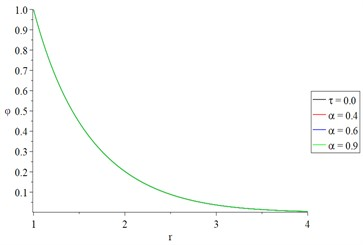a) The conductive temperature increment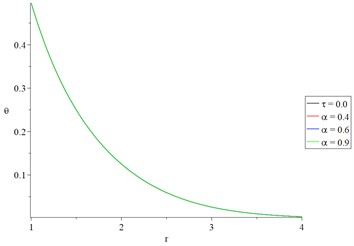b) The dynamic temperature increment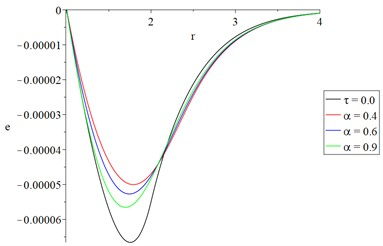c) The volumetric strain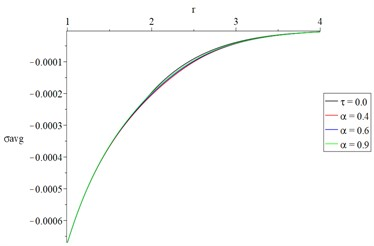d) The average of principal stresses

Fig. 2(a), 3(a), 2(b), and 3(b) represent the conductive and dynamical temperature increments, respectively. It is noted that the value of the fractional-order strain parameter has a very limited effect on the thermal wave.

Figs. 2(c) and 3(c) represent the volumetric strain, and it is noted that the fractional-order of strain parameter has a significant effect where the absolute value of the peak point of the volumetric strain increases when the value of the fractional-order strain parameter increases. Moreover, the maximum absolute value of the volumetric strain occurs when the consideration of the fractional-order strain does not exist.

Figs. 2(d) and 3(d) represent the average value of the principal stresses, and it is noted that the fractional-order of strain parameter has a small effect.

Also, we can see that the value of the dynamical temperature increment based on type-I is smaller than its value based on type-III. While the absolute value of the principal stresses based on type-I is greater than its value based on type-III.

Figs. 4 and 5 show the studied functions distributions of Green-Naghdi type-I and type-II, respectively, based on one-temperature and hyperbolic two-temperature models ($c=$0.0 and $c\ne$0.0) and various values of the fractional-order parameter $\alpha =$ (0.0, 0.5) at a time $t=$ 2.0 and ${t}_{0}=$ 2.0 to sand on the effect of the fractional-order parameter on the two studied models.

Figs 4(a), 4(b), 5(a), and 5(d) show that the hyperbolic two-temperature parameter has significant effects on the conductive and dynamical temperature increment or the thermal wave in general for the two studied types I and III.

Fig. 3The studied function distributions of Green-Naghdi type-III with various values of the fractional-order parameter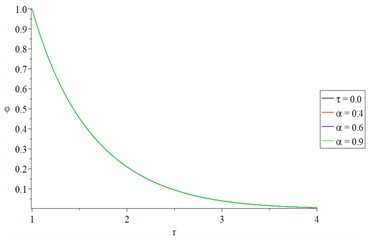a) The conductive temperature increment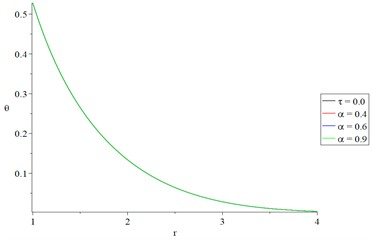b) The dynamic temperature increment.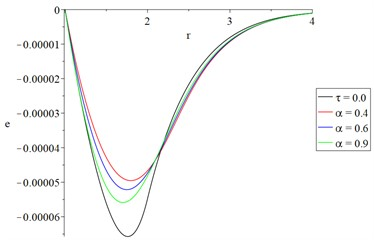c) The volumetric strain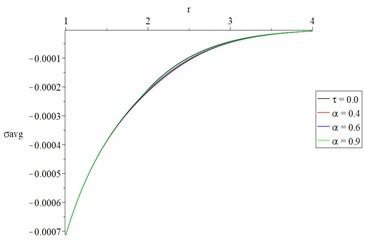d) The average of principal stresses

Fig. 4The studied functions distributions of Green-Naghdi type-I based on one-temperature and hyperbolic two-temperature and various values of the fractional-order parameter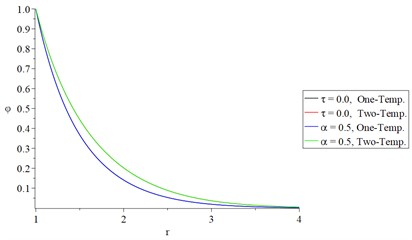a) The conductive temperature increment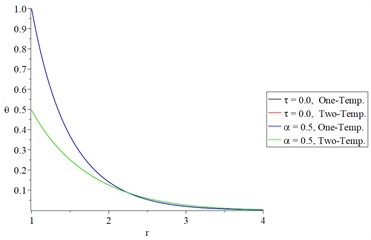b) The dynamic temperature increment.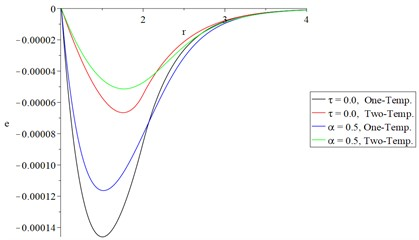c) The volumetric strain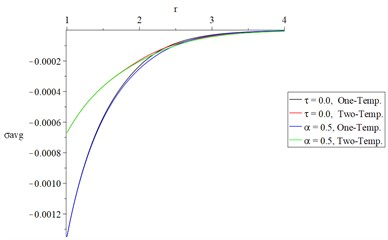d) The average of principal stresses

Figs. 4(c) and 5(c) represent the volumetric strain distributions, and it is noted that the hyperbolic two-temperature parameter has a significant effect beside the fractional-order strain parameter where the absolute value of the peak point of the volumetric strain for the two studied types take the following order:

60
${e}^{Max}\left(\tau =0.0,\mathrm{O}\mathrm{n}\mathrm{e}-\mathrm{T}\mathrm{e}\mathrm{m}\mathrm{p}.\right)>{e}^{Max}\left(\tau \ne 0,\mathrm{O}\mathrm{n}\mathrm{e}-\mathrm{T}\mathrm{e}\mathrm{m}\mathrm{p}.\right)>{e}^{Max}\left(\tau =0.0,\mathrm{T}\mathrm{w}\mathrm{o}-\mathrm{T}\mathrm{e}\mathrm{m}\mathrm{p}.\right)>{e}^{Max}\left(\tau \ne 0,\mathrm{T}\mathrm{w}\mathrm{o}-\mathrm{T}\mathrm{e}\mathrm{m}\mathrm{p}.\right)$

The above equation indicates that considering strain with fractional-order and hyperbolic two-temperature heat model leads to a decrease in the profile of the mechanical wave propagation.

Figs. 4(d) and 5(d) represent the average of principal stresses distributions, and it is noted that the two-temperature parameter has a significant effect besides the fractional-order strain parameter.

Fig. 5The studied functions distributions of Green-Naghdi type-III based on one-temperature and hyperbolic two-temperature and various values of the fractional-order parameter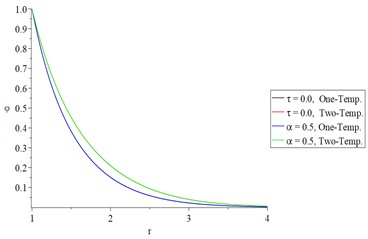a) The conductive temperature increment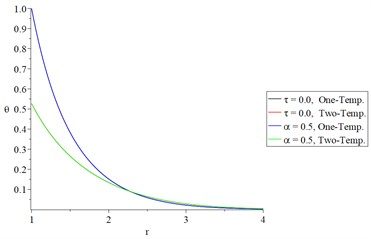b) The dynamic temperature increment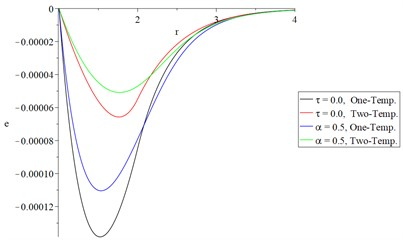c) The volumetric strain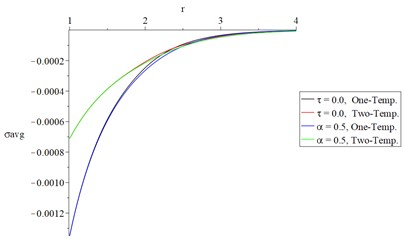d) The average of principal stresses

Figs. 6 and 7 show the studied functions distributions of Green-Naghdi type-I and type-II, respectively, based on one-temperature and hyperbolic two-temperature models ($c=$0.0 and $c\ne$0.0) and the fractional-order parameter $\alpha =0.5$ when $t<{t}_{0}$ and $t>{t}_{0}$, respectively to stand on the effect of the ramp-time heat parameter on all the studied functions.

Figs. 6(a), 6(b), 7(a), and 7(b) show that the ramp-time heat parameter has significant effects on the conductive and dynamical temperature increments. Based on the case $t<{t}_{0}$, the profile of the thermal wave’s propagation is smaller than its propagation based on the case $t>{t}_{0}$ in the context of one-temperature and hyperbolic two-temperature models of Green-Naghdi type-I and type-III.

Figs. 6(c), 6(d), 7(c), and 7(d) show that the ramp-time heat parameter has significant effects on the volumetric strain and average of principal stresses. Based on the case $t<{t}_{0}$, the absolute value of the volumetric strain and average of principal stresses (the profile of the mechanical wave’s propagation) are smaller based on the case $t>{t}_{0}$ in the context of one-temperature and hyperbolic two-temperature models of Green-Naghdi type-I and type-III.

Fig. 6The studied functions distributions of Green-Naghdi Type-I and type-III based on one-/two-temperature when α=0.5 and t<t0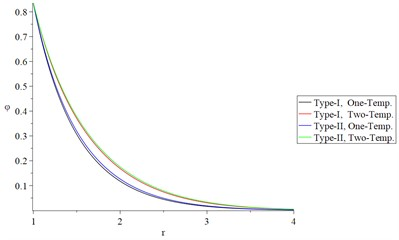a) The conductive temperature increment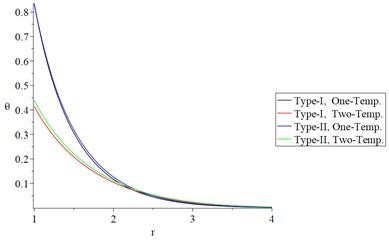b) The dynamic temperature increment.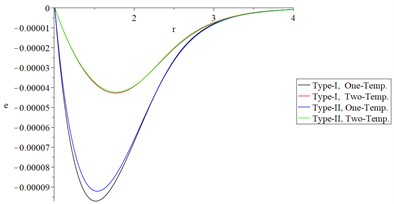c) The volumetric strain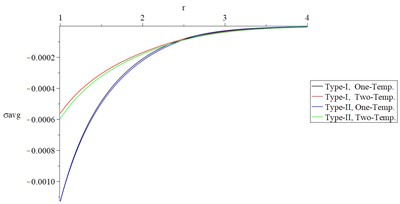d) The average of principal stresses

Fig. 7The studied functions distributions of Green-Naghdi Type-I and type-III based on one-/two-temperature when α=0.5 and t>t0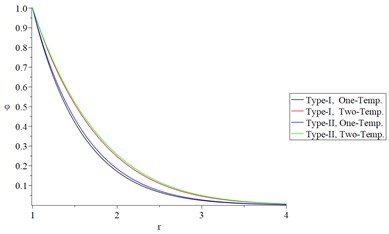a) The conductive temperature increment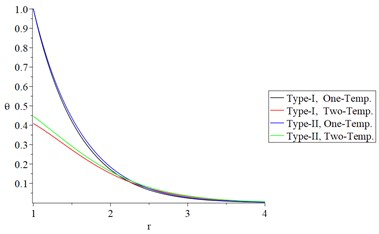b) The dynamic temperature increment.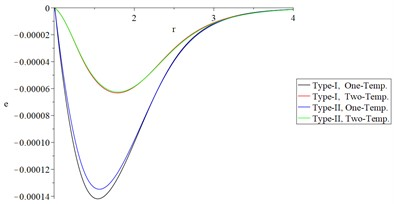c) The volumetric strain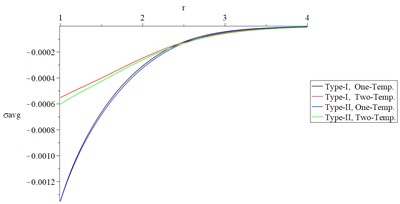d) The average of principal stresses

## 6. Conclusions

1) The value of the fractional-order strain parameter has limited effects on the conductive and dynamical temperature increments.

2) The value of the fractional-order strain parameter has significant effects on strain and stress.

3) The hyperbolic two-temperature parameter has significant effects on the conductive and dynamical temperature increments, strain, and stress.

4) The ramp-time heat parameter has significant effects on the conductive and dynamical temperature increments, strain, and stress.

5) The profile of the thermal wave’s propagation based on Green-Naghdi type-I is smaller based on Green-Naghdi type-III.

6) The profile of the mechanical wave’s propagation based on Green-Naghdi type-I is greater based on Green-Naghdi type-III.

7) Considering strain with a fractional-order and hyperbolic two-temperature heat conduction model causes the profile of mechanical wave propagation to be reduced.

8) Green-Naghdi type-I and type-II based on hyperbolic two-temperature heat conduction models offer propagation of thermal and mechanical waves with finite speeds.

04 May 2023
Accepted
08 June 2023
Published
26 June 2023
Keywords
hyperbolic two-temperature
Green-Naghdi
spherical cavity
fractional-order strain
ramp-type heat
Acknowledgements

The authors have not disclosed any funding.

Data Availability

The datasets generated during and/or analyzed during the current study are available from the corresponding author on reasonable request.

Author Contributions

Youssef construct the model, consider the conditions, obtain the numerical solutions, review all the work, and submit for publishing. Alghamdi figure the numerical results, discuss the results, set the conclusion, and write all the work.

Conflict of interest

The authors declare that they have no conflict of interest.